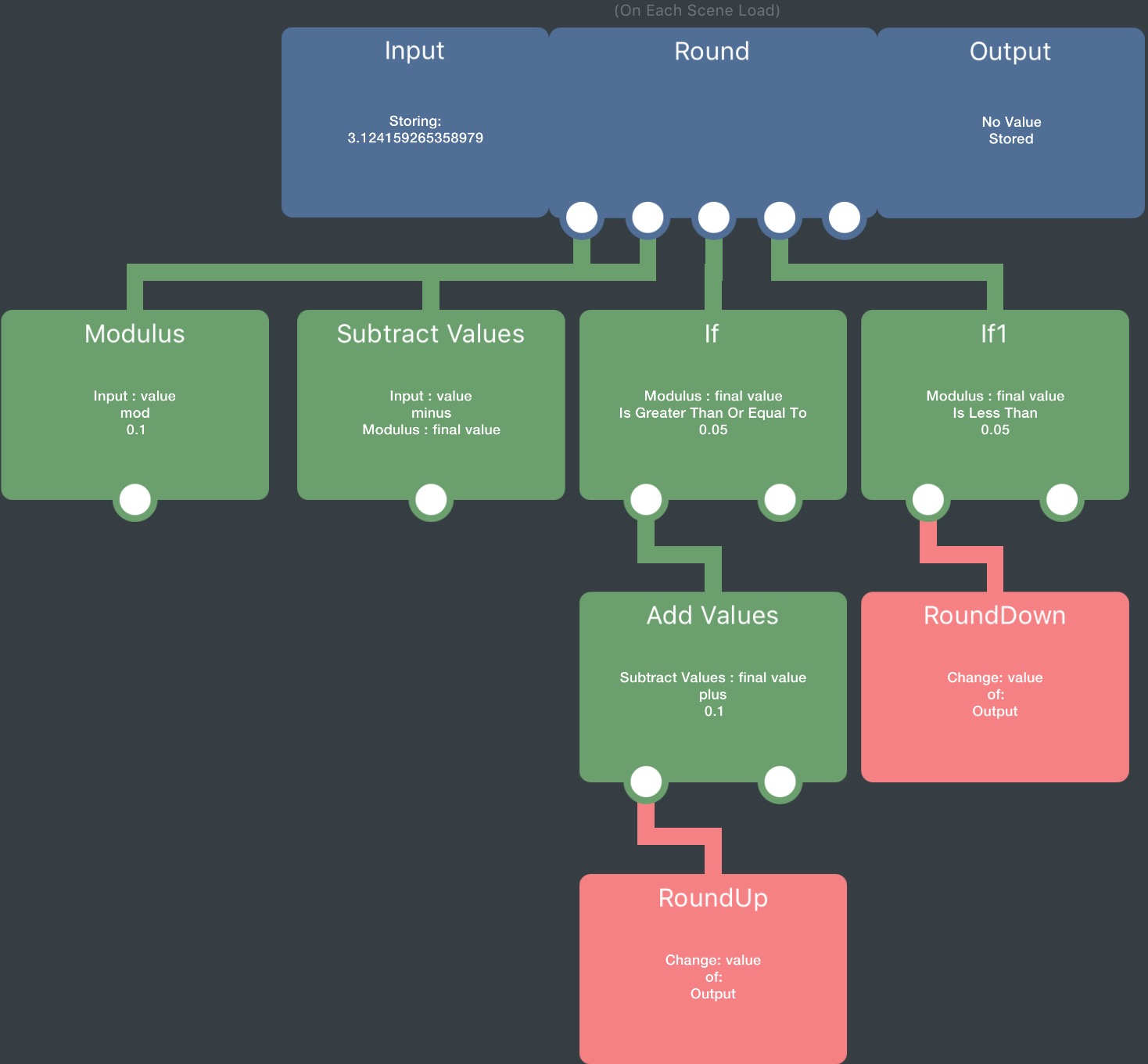Round not working...

• Re: Overlays
@Hamed i sent you new copy of the project.....I've run into a new problem😩 in the title I've added a bunch of new behaviors to set all my labels to various things...the problem is that the rounding isn't working right. Sometimes it is fine but sometimes I get a HUGE decimal. If you can't get the default location to do it, you can search a new location with the 🔎.

• @Hamed or @Murtaza did you find out why this is happening?

• I beleive @Hamed is looking into this still. I'll remind him about it tomorrow.

• @Murtaza great, thanks!

• I think its because you're dividing the number after you're rounding it. Divide first, then round.

• @Hamed the reason I'm rounding first, is because I'm trying to round it to 1 decimal place.
That's why I multiplied by ten, then rounded, then divided by 10.
For example 10.25*10 = 102.5 round that and you get 103.0 divide that by 10 and get 10.3

• I think you're going to need another round then. The math behaviours convert the number to a decimal number which is why you're seeing this behaviour.

• @Hamed but round(10x)/10 should result in one decimal digit, shouldn't it? I've used round like this before and not had a problem with it.

• @Hamed why would dividing by ten give it a decimal...it should just move the decimal place but not give it .999999999 or something, right?

• @iTap-Development Lol I get this sort of problem too with multiplication/division, get things like .999999999 or .000000001

• @iTap-Development you could try using the modulus behaviour instead of round, like this:It doesn't use multiplication or division, so you shouldn't get that problem, I don't know how useful this is though if you have to use a lot of round numbers.

• @Jack8680 yeah I do have to round a lot of numbers, but I could just have the input be an attribute that gets set to the number that needs to be rounded to reuse all the same behaviors.

• If anyone is interested, it works to get the text length of the rounded number, and then add 1 to it(since after dividing there's a decimal) and then trimming any decimals off by putting the added value as the end index and having it start from beginning. I guess depending on what you're doing with the rounded number you'll have to add or subtract or whatever from the text length.

I think i fixed the decimals in my weather app, but if anyone still has a decimal in it, please let me know!